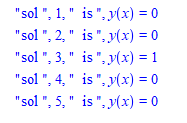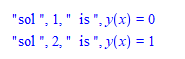# Question:an efficient way to remove objects from list, if one field is the same?

## Question:an efficient way to remove objects from list, if one field is the same?

Maple 2021

I have a list of objects. Each object contains many fields, one of these fields happened to be a solution to an ode.

At the end of solving the ODE, I'd like to remove all objects which contain the same solution.

I can not do the normal trick in Maple to remove duplicates from a list, which is to convert the list to a set and let Maple automatically remove duplicates because in this case each entry in the list is an object of many fields and hence each object is always different.

I only want to remove the objects from the list which has the same specific field.

Currently what I do is the following:   Do a first scan, and loop over all entries in the list to find the duplicates. Each time I find a duplicate solution, mark a field in the object as it is being duplicate.

Then do another scan at the end, to build a new list, which only adds those objects not marked as duplicates.

It works, but was wondering if Maple or someone might have better suggestion or more elegent way to do this.

In this MWE I am using a list and adding objects to it making it grow dynamically. I know it would be better to use Array, but for now I'd like to stick to a list, since the number of solutions is normally small. May be in the future I will change the list to Array to avoid building a list dynamically.

This example code builds a list of 5 objects

```restart;
ode_solution_type:=module()
option object;
export sol:=NULL; #only ONE solution here.
export stuff;
export mark_to_delete:=false;
end module:

SOL::list:=[]:  #where to keep the objects

for n from 1 to 5 do
tmp:=Object(ode_solution_type);

if n=3 then
tmp:-sol:=y(x)=1;
else
tmp:-sol:=y(x)=0;
fi;
tmp:-stuff:=rand();
SOL:=[op(SOL),tmp];
od:
```

In the above, I made 4 objects to have same solution which is y(x)=0 and one different. The goal is to remove all objects from the list which has duplicate solutions and keep only one copy.

```for n from 1 to numelems(SOL) do
print("sol ", n , "  is ", SOL[n]:-sol);
od:
```And this is how currently I remove the duplicates

```for n from 1 to numelems(SOL) do
for m from n+1 to numelems(SOL) do
if is(SOL[n]:-sol=SOL[m]:-sol) and not SOL[m]:-mark_to_delete then
print("found duplicate at ",m);
SOL[m]:-mark_to_delete:=true;
fi;
od;
od:

#now make new pass to keep the non- duplicates
new_SOL::list:=[]:
for n from 1 to numelems(SOL) do
if not SOL[n]:-mark_to_delete then
new_SOL:=[op(new_SOL),SOL[n]];
fi;
od:
```

checking:

```for n from 1 to numelems(new_SOL) do
print("sol ", n , "  is ", new_SOL[n]:-sol);
od:
```Does there exist a better option in Maple (while still using a list?) to remove objects in list which have the same specific field?

Could you suggest a better method?﻿